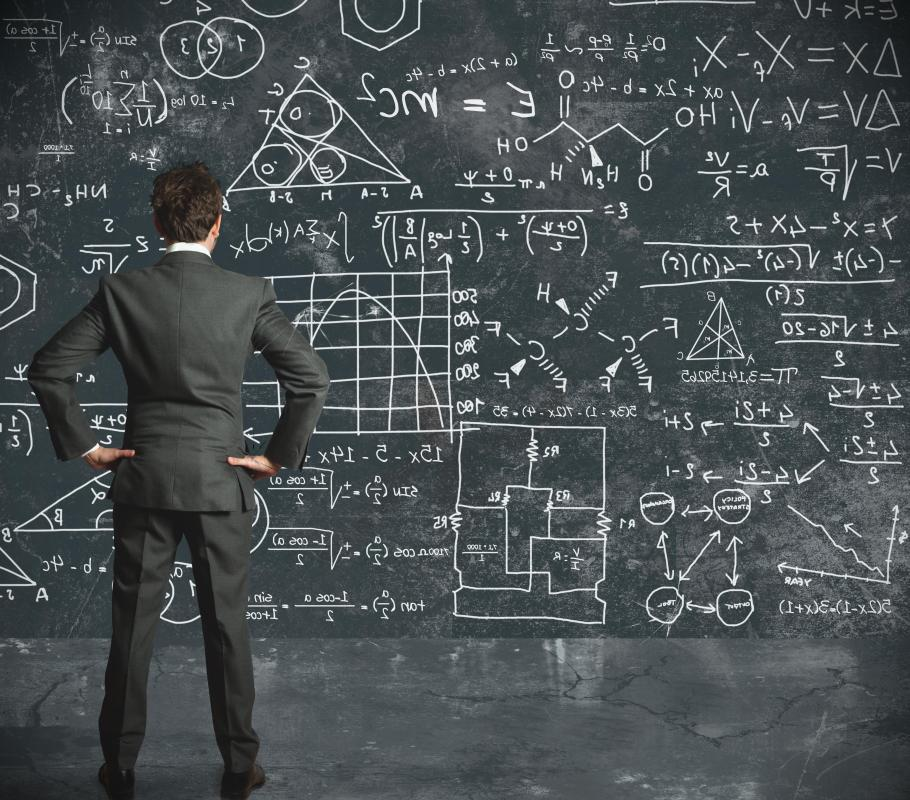# What is Potential Energy?

Daniel Liden

Potential energy is the stored energy of position. It can be thought of as energy that is “stored” by any physical system. It is called potential because, in its current form, it is not doing any work or causing any change in its surroundings. It does, however, have the potential to be converted to different forms of energy, such as kinetic energy. The standard unit for measuring such energy is the joule.

When an object is displaced from its original position and there is energy pulling it back to that position, potential energy tends to exist. A ball at the end of a spring, for example, has energy that will be converted to kinetic energy when allowed to return to its original position. A weight held above the ground will, when released, have potential energy as gravity pulls it back to its original position.Swinging on a swing is a good example of both potential and kinetic energy.

One of the major principles of this type of energy is the law of conservation of energy, which states that energy can neither be created nor destroyed. The energy expended to lift an object or compress a spring does not simply disappear, it is “stored” as potential energy. It is then released as kinetic energy by a restoring force. The energy input equals the energy output; there is no gain or loss in overall energy.

There are many different types of potential energy. Potential elastic energy exists when an elastic object, such as a bow or rubber band, is stretched or otherwise deformed under stress. Potential chemical energy is related to the chemical bonds in a molecule. In chemical reactions, chemical potential energy is transformed to other forms of energy as the bonds are broken and reformed. Potential electrical energy takes three primary forms: electrostatic, electrodynamic, and nuclear.

Potential electrostatic energy exists when a charged particle is at rest. It has potential energy because of its position in relation to other charged particles. Potential electrodynamic energy exists because of moving charged particles. These particles can form an electromagnetic field that has the potential to move other objects. Potential nuclear energy exists because of the relationships between subatomic particles, such as protons, electrons, and neutrons, in the nucleus of atoms.The formula for potential energy that is used depends on the type of energy that is stored.

## You might also Likeanon282596

What I think is that when the body is at a certain height from the ground, then it has a potential energy, as the formula for it says p.e = mgh. Since at the ground the height of the object such as rock is 0 therefore the potential energy is 0, so as mentioned above, when you lift the rock, h has a value greater than 0, so potential energy exists.

anon281556

What is potential energy? It does not state information on here that kids will understand!

jamestaylor2

It's a great article, but I'm still confused. Does an object which is at rest on the ground--such as a rock--have potential energy? If it does not, why would a book on a shelf (at rest) have potential energy? Would it be because for whatever reason it could still fall?

anon85985

potential energy is very confusing, because when you're having a test on science (e.g., potential energy, kinetic energy, force, speed) and all of these questions can come from making mouse traps expertly when you're in year 10 in high school, there's a lot of studying.

liden

Potential energy can be very confusing. Potential energy exists in essentially everything, though. For example, two subatomic particles experience potential energy just because of their relationships to each other.

If you pick up a rock and throw it, there are several different sources of energy exerted on it. You give it energy by throwing it -- your body converts chemical energy into mechanical energy to allow you to throw it. The rock has potential energy because it is above the ground. The potential energy is equal to its mass times the height above the ground times the acceleration due to gravity.

When it is actually thrown, the chemical/mechanical energy from you and the potential energy that exists because of gravity are converted to kinetic energy, the energy of motion.

A blade of grass is a more complex case. While potential energy is at work, the wind's energy is much more important; the effects of potential energy are essentially negligible.

breakofday

Man this is confusing. So basically, does EVERYTHING store potential energy?

A rock on the ground has potential energy IF I pick it up and throw it, which is then converted to Kinetic energy. But wouldn't that my MY potential energy that is being expended and converted to Kinetic energy and not the rocks?

Or how about a blade of grass on the wind, does the GRASS have the potential energy or is it the wind blowing thereby using its' Kinetic energy to move the grass?